Here you will learn about sort.

In Bubble Sort, all we have to do is pick the first two elements of the array and compare them if first one is greater than the second one then swap them. After it, pick next two elements and compare them and so on.

After travelling the array once, the greatest number will be placed on the last index.

To sort entire array we have to travel the array (n-1) times where n is the length of the array.

## Python Bubble Sort

### Example

Let’s  consider we have an array:

21,14,18,25,9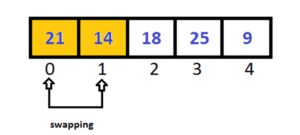Pass-1:

As we know 14 is less than 21 so swap them and pick next two elements.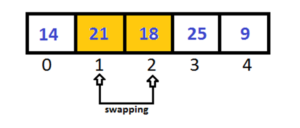Again 18 is less than 21, so we have to swap them, and pick next two elements.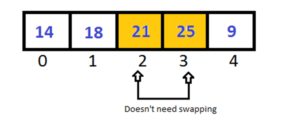25 is greater than 21 so we doesn’t need to swap them, now pick next two elements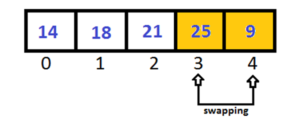9 is less than 25, swap them.So here we have been traveling array once and the largest number is placed at last index.

As we mentioned above that to sort the entire array we have to travel array (n-1) times, where n is the length of array.

So we name it Pass-1.

Let’s start Pass-2.

Pass-2:

This time we doesn’t need to check the last index, because it is sorted after Pass-1.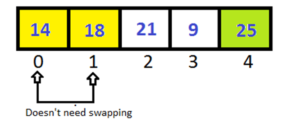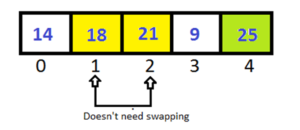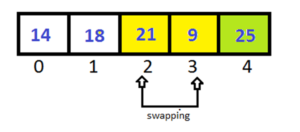After completing Pass-2 the last two elements have been sorted. So in Pass-3 we don’t have to check them.

Pass-3: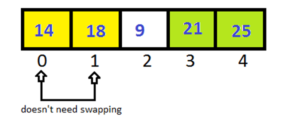After completing Pass-3 , the last 3 elements of array are in sorted order.

Pass-4: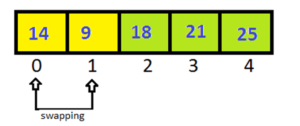We have  been traveled array (n-1) times. Array has been sorted.

As after every step the largest element tend to move up into the correct order like bubbles rising to the surface, that’s why it is called as BUBBLE SORT.”

### Algorithm

we have an array Arr[n], where n is number of elements.

Step:1-  set pass =1

Step:2- set index = 0

Step:3- if Arr[index]>Arr[index+1]

Then swap Arr[index] with Arr[index+1]

Increase index by 1.

Step:4- Go to Step:3 till index<=n-pass-1

[pass will prevent  checking of the sorted element again]

Step:5- increase pass by 1 and go to Step:2 till pass<=n-1

Output

9 14 18 21 25

SHARE